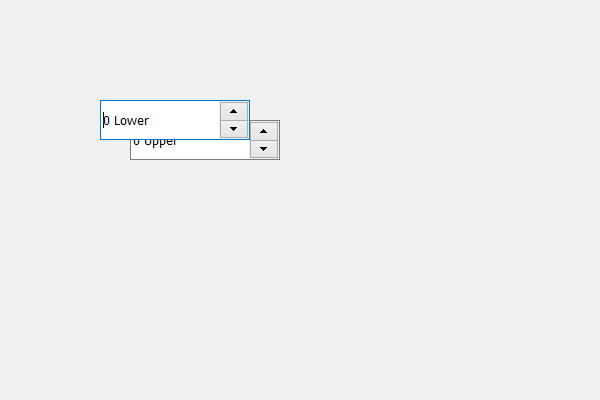GFG App
Open AppBrowser
Continue

# PyQt5 QSpinBox – Showing it on above level

In this article we will see how we can make the spin box at above level, above level means at it will show on the top if it collides with some other spin box position, by default when we create two spin box at similar position, the spin box which was created at the end will show on the top although we can change this.

In order to do this we will use `raise_()` method.

Syntax : spin_box.raise_()

Argument : It takes no argument

Return : It returns None

Note : This method is used with the spin box which was created earlier

Below is the implementation

 `# importing libraries ` `from` `PyQt5.QtWidgets ``import` `*`  `from` `PyQt5 ``import` `QtCore, QtGui ` `from` `PyQt5.QtGui ``import` `*`  `from` `PyQt5.QtCore ``import` `*`  `import` `sys ` ` `  `class` `Window(QMainWindow): ` ` `  `    ``def` `__init__(``self``): ` `        ``super``().__init__() ` ` `  `        ``# setting title ` `        ``self``.setWindowTitle(``"Python "``) ` ` `  `        ``# setting geometry ` `        ``self``.setGeometry(``100``, ``100``, ``600``, ``400``) ` ` `  `        ``# calling method ` `        ``self``.UiComponents() ` ` `  `        ``# showing all the widgets ` `        ``self``.show() ` ` `  `    ``# method for widgets ` `    ``def` `UiComponents(``self``): ` ` `  `        ``# creating spin box ` `        ``self``.spin ``=` `QSpinBox(``self``) ` ` `  `        ``# setting geometry to spin box ` `        ``self``.spin.setGeometry(``100``, ``100``, ``150``, ``40``) ` ` `  `        ``# setting suffix to spin ` `        ``self``.spin.setSuffix(``" Lower"``) ` ` `  `        ``# creating spin box ` `        ``self``.spin_upper ``=` `QSpinBox(``self``) ` ` `  `        ``# setting geometry to spin_upper ` `        ``self``.spin_upper.setGeometry(``130``, ``120``, ``150``, ``40``) ` ` `  `        ``# setting suffix to spin_upper ` `        ``self``.spin_upper.setSuffix(``" Upper"``) ` ` `  `        ``# making spin rise above ` `        ``self``.spin.raise_() ` ` `  ` `  `# create pyqt5 app ` `App ``=` `QApplication(sys.argv) ` ` `  `# create the instance of our Window ` `window ``=` `Window() ` ` `  `# start the app ` `sys.exit(App.``exec``()) `

Output :My Personal Notes arrow_drop_up